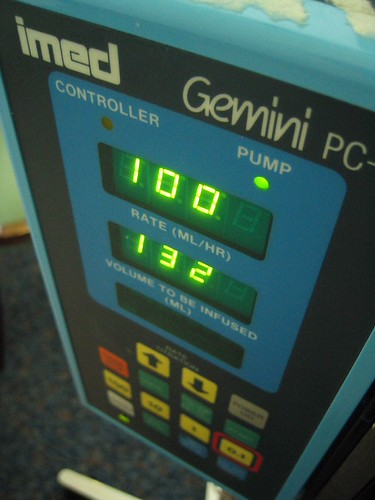# My Dominant Hemisphere

The Official Weblog of 'The Basilic Insula'

## Infusions Redux, DNS And Cerebral EdemaThere’s a book on fluid and electrolyte management that I’ve been reading recently. Called, “Practical Guideline on Fluid Therapy” and authored, as probably evident by the English used in the title, by a very Indian Sanjay Pandya, the book contains many interesting nuggets for day to day practice. Although like most Indian books there is a distinct absence of the emphasis on applying one’s brain, it is nevertheless worth the time to peruse. Today I will be discussing two equations from the book and a question that came up in my mind about the usage of a specific fluid.

### Calculating ECF volume deficit (in dehydration, etc.)

1. If the patient’s previous body weight is known, all you gotta do to obtain ECF deficit is find out the difference between his present and past weight.
2. Another technique uses changes in the Hematocrit to discern ECF volume deficit. This method is applicable only if there is no hemorrhage, hemolysis or other situations involving loss of blood cells, the idea being that any change in blood volume is caused by plasma volume change. So if there’s dehydration and loss of ECF volume, plasma volume shrinks and causes the hematocrit to rise.

ECF Volume Deficit in liters = 0.2 * lean body weight * [(Current hematocrit/Desired hematocrit) – 1]

Can someone figure out the proof for the above equation and post it here? Like most other stuff, I absolutely hate roting math formulas and prefer remembering their derivations. This equation is taking me some time to prove.

To help get started, here are a couple of possible pointers I’m currently exploring:

Total body water (TBW) when expressed as a percentage of Total body weight (TBwt), varies by gender and age. In young adult men for example

TBW = 60% TBwt

TBW in liters

TBwt in kg

Interestingly enough, TBW when expressed as a percentage of lean body weight (LBwt) is a constant and isn’t conditioned upon gender or age.

TBW = 70% LBwt

LBwt = (100/70) * TBW

= (100/70) * [(x/100) * TBwt]

= (x/70) * TBwt

x is the percentage of TBwt that is TBW

Plasma volume is related to blood volume as follows

Plasma volume = Blood volume * [(100 – Hematocrit)/Hematocrit]

Plasma volume is also 1/4 of ECF volume. ECF is 1/3 of TBW. So plasma volume is 1/12 of TBW.

### Calculating Electrolyte Infusion Rates

Change in plasma electrolyte concentration in mEq/L when 1 liter of  infusate is given

= [Infusate electrolyte concentration in mEq/L – Actual electrolyte concentration in mEq/L] / (TBW + 1)

This one’s easy to derive. Taking Na+ as our electrolyte example,

Initial Na+ content = x * TBW

Initial Na+ concentration = (x * TBW)/TBW

Final Na+ content after infusing 1L infusate = (x * TBW) + {y * 1}

Final Na+ concentration = [(x * TBW) + {y}]/(TBW + 1)

Change in Na+ concentration due to infusion = [(x * TBW) + {y}/(TBW + 1)] – [(x * TBW)/TBW]

= (yx)/(TBW+1)

x = mEq/L of Na+ initially in the body

y = mEq/L of Na+ in the infusate

And voila! There you have it!

And now for that promised question:

Given the fact that DNS (Dextrose Normal Saline) only stays in the ECF, would it be right to assume that it’s contraindicated in cerebral edema?

The interesting thing is that on exploring the scientific literature, I found that recent research shows that it isn’t just the shifting of fluid into the brain parenchyma that should be avoided when infusing fluid; hyperglycemia is a real danger as well. How hyperglycemia contributes to cerebral edema and especially in situations of cerebral ischemia is a topic of ongoing research and multiple plausible hypotheses are being investigated.

As per Pandya’s book, by the way, it is best to restrict glucose infusion to ≤ 0.5 grams/kg/hour when infusing any glucose containing fluid to avoid complications of hyperglycemia.

Kincaid: 11.4
ARI: 12.4
Coleman-Liau: 11.2
Flesch Index: 57.0/100
Fog Index: 14.6
Lix: 46.9 = school year 8